# 正态分布变换(The Normal Distributions Transform)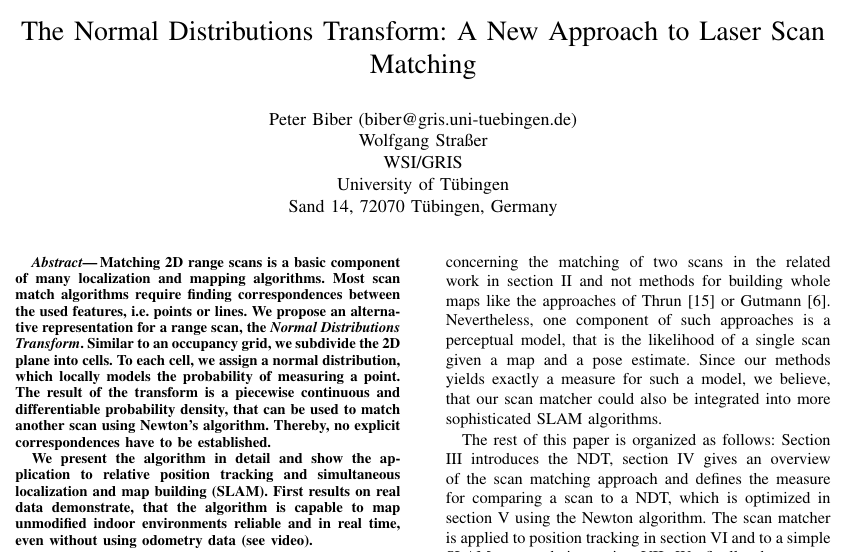## 算法提出

Biber和Straer提出了二维数据配准的正态分布变换(NDT)方法。该算法中的关键元素是参考扫描的表示。不是将当前扫描直接匹配到参考扫描的点，而是通过正态分布的线性组合来模拟在特定位置找到表面点的可能性。

• 点云配准
• 地图匹配

## 其他配准算法

1. ICP

• 要剔除不合适的点对（点对距离过大、包含边界点的点对）
• 基于点对的配准，并没有包含局部形状的信息
2. IDC

• ICP的一种改进，采用极坐标代替笛卡尔坐标进行最近点搜索匹配
3. PIC

• 考虑了点云的噪音和初始位置的不确定性
4. Point-based probabilistic registration

5. Gaussian fields

• 和NDT正态分布变换类似，利用高斯混合模型考察点和点的距离和点周围表面的相似性
6. Likelihood-field matching——随机场匹配

7. CRF匹配

8. Branch-and-bound registration

9. Registration using local geometric features

# 2D-NDT基本原理

NDT算法论文原文是从2D角度描述的。

## 概率密度计算

NDT利用局部正态分布建立了一次激光扫描所有重建二维点的分布模型，首先，将机器人周围的二维空间细分为固定大小的网格(类似于占据栅格地图)，然后对于每一个单元，进行下面3个操作

• 收集在这个窗口内的所有2D点
• 计算2D点集的质心$$q=\frac{1}{n}\sum_{i} x_i$$
• 计算协方差矩阵$$\Sigma=\frac{1}{n}\sum_i(x_i-q)(x_i-q)^T$$

\begin{aligned} p(x) \sim \exp (-\frac{(x-q)^T \Sigma^{-1}(x-q)}{2}) \end{aligned}

• 为了最小化离散化的影响，我们决定使用四个重叠的网格，首先放置一个边长为l的单网格，第二个则水平平移$$\frac{l}{2}$$长度，第三个则垂直方向平移$$\frac{l}{2}$$长度，而最后一个则水平和垂直都平移$$\frac{l}{2}$$长度。如下图所示，现在每个2D点都落入到这4个cell里面，因此，如果计算一个点的概率密度，即对所有四个单元的密度进行评估，并对结果进行求和。但是在下面的讨论中，暂时不考虑这样的情况，只需考虑只有1个cell即可。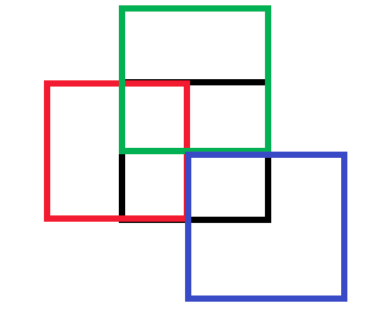• 另外一个点就是，如果在一个完全没有噪声的理想情况下，协方差矩阵会变成奇异的，无法求逆。在实际情况中，协方差矩阵有时候十分接近奇异，为了避免这种情况，进行检查，检查协方差矩阵的最小特征值是否至少是最大特征值的0.001倍，如果不是，则设置该值。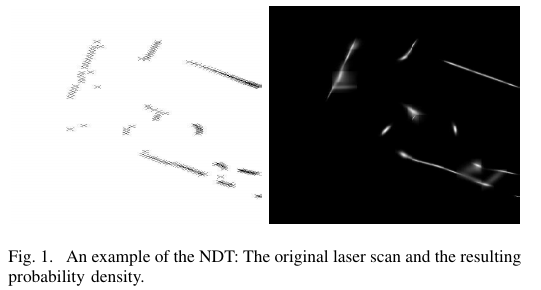## 帧间配准(匹配)

1. 对第一帧进行NDT处理
2. 参数(R,t)初始化，可设置为0或者使用里程计的数据
3. 对于第二帧的每一个样本，利用待优化参数将第二帧的点反投影到第一帧的坐标系中
4. 确定每个映射点对应的正态分布 ？
5. 参数(R,t)的得分是通过评估每个映射点的分布并对结果求和来确定的
6. 计算一个新的参数估计试图优化评分。这是通过执行牛顿算法的一个步骤完成的
7. 重复，直到收敛

• 待优化参数$$\boldsymbol{T}()=\boldsymbol{P}=(p_i){i=1,2,3}=(t_x,t_y,\phi)^T$$
• 第二帧的第i个扫描点$$x_i$$
• 第二帧的第i个扫描点反投影回第一帧坐标系后得到的点$$x_i'=T(x_i,\boldsymbol{P})$$
• $$\Sigma_i,q_i$$分别是第二帧反投影点$$x_i'$$的联合高斯分布的协方差矩阵和均值，在第一帧的NDT变换中查找

$score(p)=\sum_i \exp ( \frac{-(x_i'-q_i)^T \Sigma_i^{-1}(x_i'-q_i)}{2})$

## 牛顿迭代法优化

$\boldsymbol{H\Delta p}=-\boldsymbol{g}$

• $$\boldsymbol{g}$$是函数$$f()$$的梯度转置，也就是雅克比
• $$\boldsymbol{H}$$是对应的海森矩阵

$\boldsymbol{p} \leftarrow \boldsymbol{p} +\Delta \boldsymbol{p}$

$-score= s =- \exp \frac{- \boldsymbol{q^T} \Sigma^{-1} \boldsymbol{q} }{2}$

\begin{aligned} \bar{g_i} &= - \frac{ \partial s}{ \partial p[i]}= - \frac{ \partial s}{ \partial q} \frac{q}{p[i]} \\ &= \boldsymbol{q^T} \Sigma^{-1} \frac{ \partial q}{ \partial p[i]} \exp \frac{- \boldsymbol{q^T} \Sigma^{-1}q}{2} \end{aligned}

\begin{aligned} u' = \cos (p) u -\sin(p) v+ p\\ v' = \sin (p) u -\cos(p) v + p \end{aligned}

\begin{aligned} \boldsymbol{J_T}= \frac{\partial \boldsymbol{q} }{\partial \boldsymbol{T} }=\frac{\partial \boldsymbol{q} }{\partial \boldsymbol{P} }= \begin{pmatrix} 1 & 0 & -x \sin \phi -y \cos \phi \\ 0 & 1 & x \cos \phi - y \sin \phi \end{pmatrix} \end{aligned}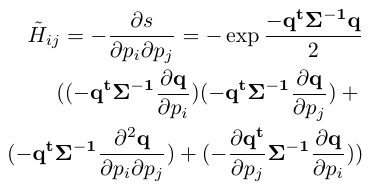\frac{ \partial ^2 \boldsymbol{q} }{ \partial p_i \partial p_j}= \left \{ \begin{aligned} \begin{pmatrix} -x \cos \phi + y \sin \phi \\ -x \sin \phi - y \cos \phi \end{pmatrix} ~~ i=j=3 \\ \begin{pmatrix} 0 \\ 0 \end{pmatrix} ~~~~~~~~~~~~~~~ otherwise \end{aligned} \right.

# 应用于位置跟踪

• 设置一个从$$t_k-1$$$$t_k$$的运动估计作为初值$$\delta$$
• 将当前帧的激光扫描点根据这个$$\delta$$反投影到上一个时刻的机器人坐标系下
• 使用当前帧的扫描、参考关键帧的NDT以及新的运动估计$$\delta$$来实现优化迭代
• 检查参考关键帧与当前帧是否比较靠近，如果是，则继续迭代，否则，采用上一次成功匹配的激光扫描作为新的关键帧。

# SLAM上的应用

## 添加关键帧以及优化地图

$socre'(\Delta T)= score + \frac{1}{2} (\Delta T)^T H (\Delta T)$

## 文章实现效果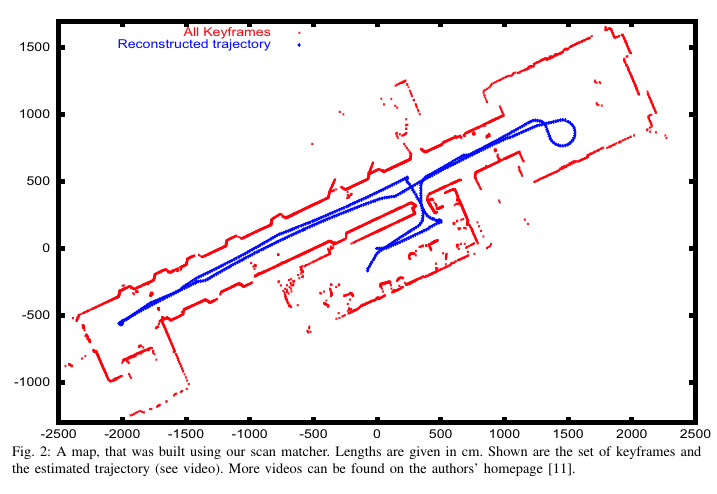## 2020-03-06更新： PCL中关于2D-NDT的代码及注释

PCL代码基本与论文一致

ndt_2d.h

ndt_2d.hpp